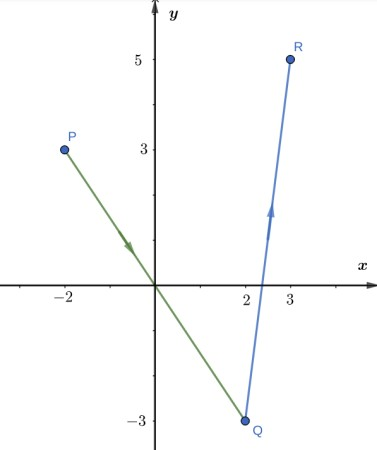# Give a set of parametric equations that describes the following curves. Graph the curve and...

## Question:

Give a set of parametric equations that describes the following curves. Graph the curve and indicate the positive orientation. If not given, specify the interval over which the parameter varies.

The piecewise linear path from P(-2,3) to Q(2,-3) to R(3,5), using parameter values {eq}0 \geq t \leq 2. {/eq}

## Parametric Line Segments

To find a parametrization of a line segment between two given points {eq}\displaystyle A(x_0,y_0), {/eq} {eq}\displaystyle B(x_1,y_1) {/eq}

we will determine the direction vector {eq}\displaystyle \mathbf{AB}=\langle x_1-x_0, y_1-y_0\rangle {/eq} of the line and

set up the starting and ending values of the parameter from the parametrization,

by taking multiples of the direction vector {eq}\displaystyle a\langle x_1-x_0, y_1-y_0\rangle. {/eq}

So, a parametrization with {eq}\displaystyle t=0 \text{ corresponding to }A {/eq} the starting point, will look like {eq}\displaystyle x=x_0+at(x_1-x_0), y= y_0+at(y_1-y_0), 0\leq t\leq t_{final}. {/eq}

To find a parametric vector equation for the line segment from {eq}\displaystyle P=(-2,3) \text{ to } Q=(2,3) , {/eq} such that {eq}\displaystyle 0\leq t\leq 2, {/eq}

we will first determine the direction vector of the direction line, which is

{eq}\displaystyle \vec{PQ}=\langle 4,-6\rangle =2\langle 2, -3\rangle. {/eq}

Therefore the vector parametric equation is {eq}\displaystyle \mathbf{r}(t) =\langle -2+2at, 3-3bt\rangle, {/eq} where {eq}\displaystyle a, b {/eq} are determined from the condition of the end-point {eq}\displaystyle t=2 \text{ to be } Q {/eq}

{eq}\displaystyle \begin{align}\mathbf{r}(2)&=\langle -2+4a, 3-6b\rangle =\langle 2,-3\rangle \iff \begin{cases} -2+4a=2\\ 3-6b=-3 \end{cases}\iff a=1, b=1\\ &\boxed{\text{ therefore, the parametrization of PQ is } \mathbf{r}(t)=\left\langle -2+2t, 3-3t\ \right\rangle, 0\leq t\leq 2}. \end{align} {/eq}

Similarly, for the line segment QR:

we determine the direction vector of the direction line, which is

{eq}\displaystyle \vec{QR}=\langle 1,8\rangle . {/eq}

Therefore the vector parametric equation is {eq}\displaystyle \mathbf{r}(t) =\langle 2+at, -3+8bt\rangle, {/eq} where {eq}\displaystyle a, b {/eq} are determined from the condition of the end-point {eq}\displaystyle t=2 \text{ to be } R {/eq}

{eq}\displaystyle \begin{align}\mathbf{r}(2)&=\langle 2+2a, -3+16b\rangle =\langle 3,5\rangle \iff \begin{cases} 2+2a=3\\ -3+16b=5 \end{cases}\iff a=\frac{1}{2}, b=\frac{1}{2}\\ & \boxed{ \text{ therefore, the parametrization of QR is }\mathbf{r}(t)=\left\langle 2+\frac{t}{2}, -3+4t\ \right\rangle, 0\leq t\leq 2}. \end{align} {/eq}

In the figure below, we plotted the line segments together with the direction of each parametrization,